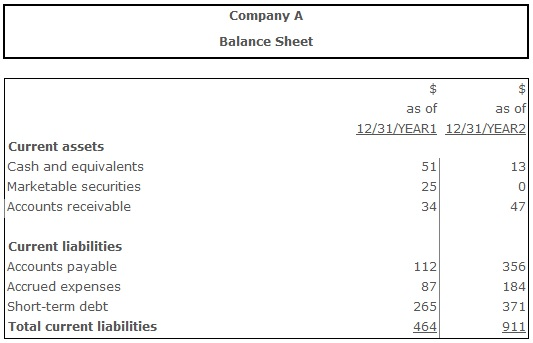# Quick Ratio

Quick Ratio (Acid Test Ratio) – an indicator of a firm’s short-term liquidity measuring how well company can meet its short-term obligations with its highly liquid assets, such as cash and equivalents, marketable securities and receivables. This ratio is similar to current ratio, as both of them measure the short-term solvency of a firm. However, quick ratio does it more rigorously, excluding the less liquid current assets and potential sources of loss from calculation. Reason for excluding these (for example, slow-moving or obsolete inventory) is need of estimating more immediate liquidity of a company than current ratio does.

The normative value of the quick ratio depends on the firm's industry and peculiarities. Most commonly quick ratio value of 1 is considered to be normal. It means that the company has as much assets with relatively good liquidity, as its current liabilities. However, to make an accurate conclusion the ratio should be compared with the dynamics of values from past periods, and with industry averages as well. Some industries find quick ratio below 1 acceptable, while for some industries only ratio above 1 is adequate. Businesses selling goods or services primarily for cash with no involvement of accounts receivable can still be on a good level of liquidity even with quick ratio moderately below 1. Conversely, if a firm has an active policy of providing buyers with consumer loans, and the accounts receivalbe turnover is slow, creating big amount of bad quality receivables, company can demonstrate unsatisfactory liquidity even with quick ratio values much greater than 1.

## Resolving the problems with the quick ratio exceeding the normative range:

If the quick ratio value is lower than normative, the firm should take some actions to increase the amount of highly liquid assets and decrease the amount of current assets.

## Formula(s):

Quick Ratio = (Cash Equivalents + Marketable Securities + Net Receivables) ÷ Current Liabilities

Quick Ratio = (Cash + Marketable Securities + Accounts and Notes Receivable) ÷ Current Liabilities

Quick Ratio = (Current assets - Inventory) ÷ Current liabilities

## Example:Quick Ratio (Year 1) = (51 + 25 + 34) ÷ 464 = 0,23

Quick Ratio (Year 2) = (13 + 0 + 47) ÷ 911 = 0,06

The quick ratio for year 1 was 0,23, meaning that there was \$0,23 of the most liquid assets for every \$1,00 of current liabilities. The decline of the ratio to 0,06 in year 2 witnessed, that the company’s short term-liquidity has become worse and it most likely would face problems paying its short-term obligations.

## Conclusion:

Quick ratio helps an analyst to estimate firm's short-term solvency and measure the amount of highly liquid assets available to cover its current liabilities, if needed. Optimal is the situation when this ratio equals 1, or close to it.